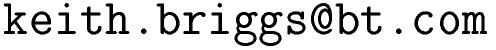Keith Briggshome · papers · thesis · talks · meetings · records · maths notes « · software · languages · music · travel · cv · students · maps · place-names · people · photos · links · ex libris · site map### Interpoint distances for two colours of points

Suppose red points form a one-dimensional Poisson spatial process with density λr. Suppose blue points are distributed similarly and independently with density λb. In the figure, λr=1 and λb=1.5.What is the probability that two neighbouring points are the same or different colours?

Let r,b be the interpoint distance between red and blue points respectively. These RVs have pdf exp(-r/λr)/λr and exp(-b/λb)/λb. Let λ=λrb. The difference d=r-b thus has pdf (here [] is the indicator function)

(exp(-d/λr)[d≥0]+exp(d/λb)[d<0])/λ.

Integrating this, we get the simple final result: if I am a red point, my nearest neighbour on the right is blue if d>0, and Prob[d>0]=λr/λ.

Therefore

Prob[two neighbours are of different colours]

=Prob[red]Prob[neighbour blue]+Prob[blue]Prob[neighbour red]

=(λr/λ)λr/λ+ (λb/λ)λb

=( λr2+ λb2 )/λ2.

This website uses no cookies. This page was last modified 2013-05-12 10:17 by.